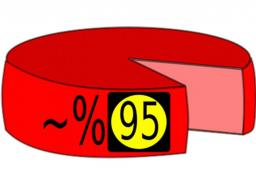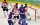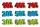# Percentages

Expressed as a percentage:

Correct result:

39/125 =  31.2 %
7/52 =  13.4615 %
43/164 =  26.2195 %
1.82 =  182 %
21/95 =  22.1053 %
40/78 =  51.2821 %
4/7 =  57.1429 %

#### Solution:We would be pleased if you find an error in the word problem, spelling mistakes, or inaccuracies and send it to us. Thank you!Tips to related online calculators

## Next similar math problems:

• Seeds 2How many seeds germinated from 1000 pcs, when 23% no emergence?
• IronIron ore contains 57% iron. How much ore is needed to produce 20 tons of iron?
• NumberWhat number is 20 % smaller than the number 198?
• Conference148 is the total number of employees. The conference was attended by 22 employees. How much is it in percent?
• GlovesI have a box with two hundred pieces of gloves in total, split into ten parcels of twenty pieces, and I sell three parcels. What percent of the total amount I sold?
• New refrigeratorNew refrigerator sells for 1024 USD, Monday will be 25% discount. How much USD will save, and what will be the price?
• Base, percents, valueBase is 344084 which is 100 %. How many percent is 384177?
• The hockeyThe hockey player scored 6 goals from 15 shots. How many % was he successful?
• Sales offGoods is worth € 70 and the price of goods fell two weeks in a row by 10%. How many % decreased overall?
• Percents - easyHow many percent is 432 out of 434?
• Apples 2James has 13 apples. He has 30 percent more apples than Sam. How many apples has Sam?
• Highway repairThe highway repair was planned for 15 days. However, it was reduced by 30%. How many days did the repair of the highway last?
• ClassIn 7.C clss are 10 girls and 20 boys. Yesterday was missing 20% of girls and 50% boys. What percentage of students missing?
• Double percentWhat is 40% of 80% of 11800?
• TVsProduction of television sets increased from 3,500 units to 4,200 units. Calculate the percentage of production increase.
• The percentages in practiceIf every tenth apple on the tree is rotten it can be expressed by percentages: 10% of the apples on the tree is rotten. Tell percent using the following information: a. in June rained 6 days b, increase worker pay 500 euros to 50 euros c, grabbed 21 from
• ClassIn a class are 32 pupils. Of these are 8 boys. What percentage of girls are in the class?